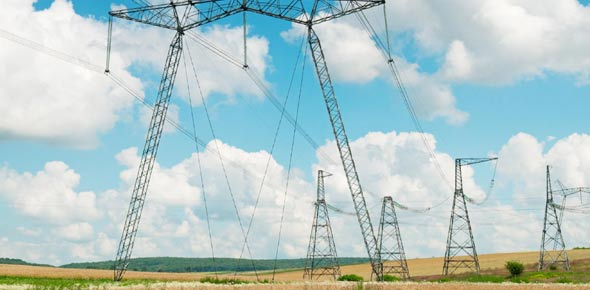# Current Electricity Quiz For 4th Grade Science Student

8 Questions | Total Attempts: 2695SettingsCurrent electricity is the constant flow of electrons and is measured by using an ammeter. This type of electricity can either be direct current or alternate current. This quiz covers the basic material taught to a 4th grade science students about current electricity. Give it a try and see just how much you remember from class these past few weeks.

Related Topics
• 1.
• A.

Circuit

• B.

Current electricity

• C.

Electron

• D.

Battery

• 2.
In a ____________ the switch is closed and electric current flows from the source through the connection device.
• A.

Open circuit

• B.

Light bulb

• C.

Closed circuit

• D.

Wire

• 3.
A _____________ was the source of electricity in the labs that we did.
• A.

Light bulb

• B.

Battery

• C.

Wire

• 4.
Benjamin Franklin was credited with inventing the light bulb.
• A.

True

• B.

False

• 5.
________ conducts electricity.
• A.

Light bulbs

• B.

Wire

• C.

Batteries

• 6.
Current electricity is a type of electricity where electrons move from one place to another through an electric current.
• A.

True

• B.

False

• 7.
A ___________________ is the object that changes electrical energy into light energy.
• A.

Light bulb

• B.

Wire

• C.

Battery

• 8.
There are four places that must be connected on the battery and bulb in order for the bulb to light.
• A.

True

• B.

False